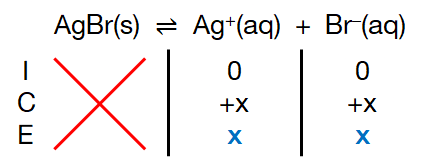# Problem: Using Appendix D, calculate the molar solubility of AgBr in(a) pure water(b) 3.0x10-2 M AgNO3 solution(c) 0.10 M NaBr solution.

###### FREE Expert Solution

Given: AgBr(s) ⇌ Ag+(aq) + Br(aq); Ksp = 5.35 × 10–13

Ksp Expression:

$\overline{){{\mathbf{K}}}_{{\mathbf{sp}}}{\mathbf{=}}\mathbf{\left[}{\mathbf{Ag}}^{\mathbf{+}}\mathbf{\right]}\mathbf{\left[}{\mathbf{Br}}^{\mathbf{-}}\mathbf{\right]}}$

(a) pure water

ICE Chart:82% (285 ratings)###### Problem Details

Using Appendix D, calculate the molar solubility of AgBr in

(a) pure water

(b) 3.0x10-2 M AgNO3 solution

(c) 0.10 M NaBr solution.